Anzeige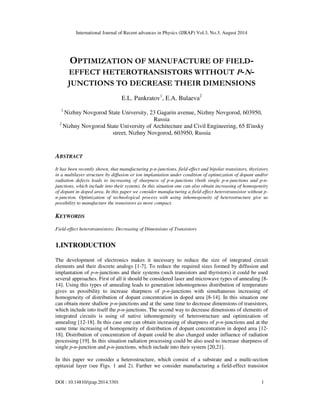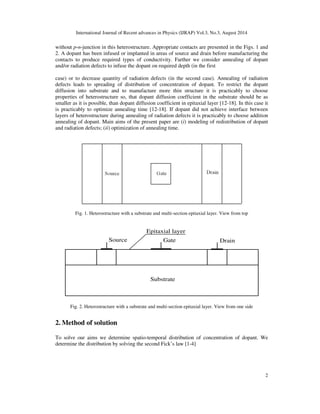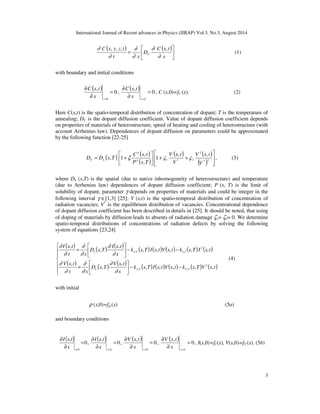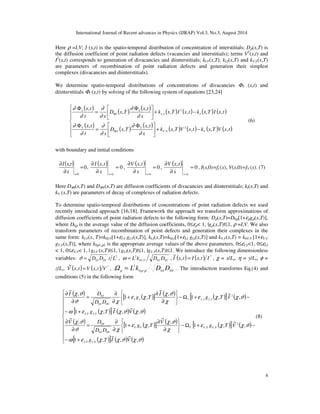Anzeige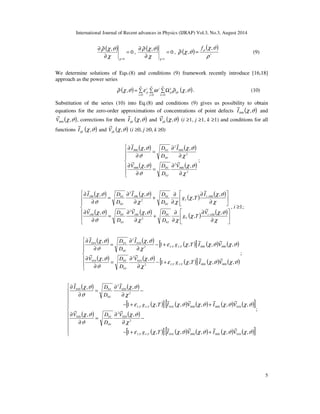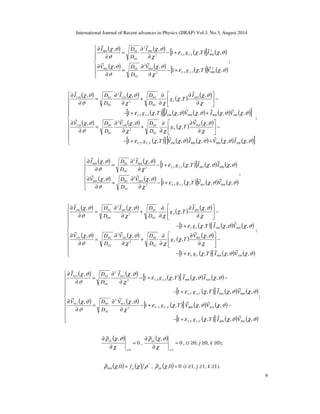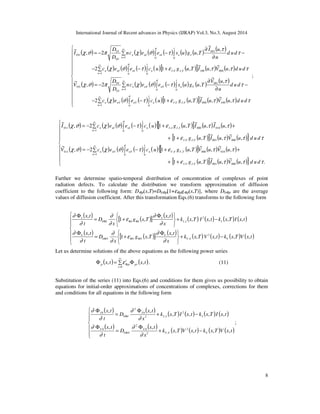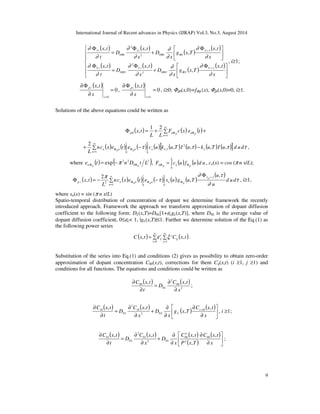Anzeige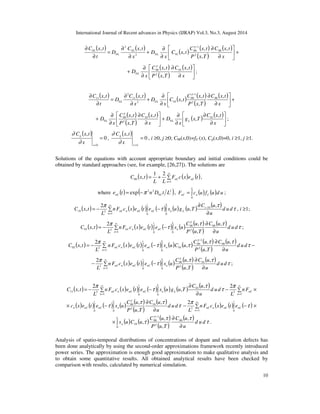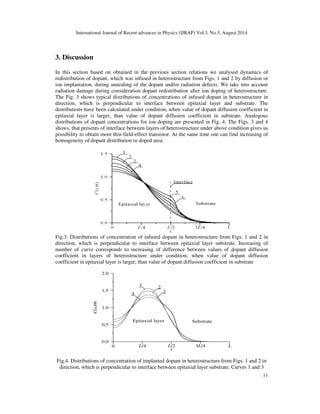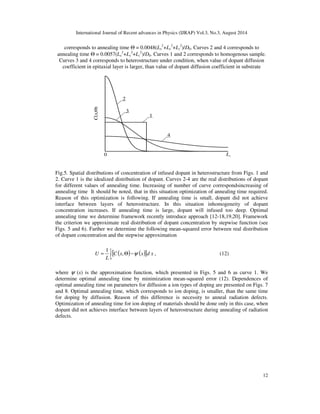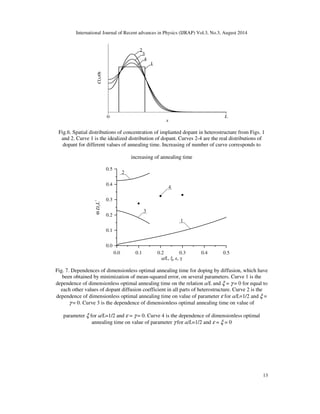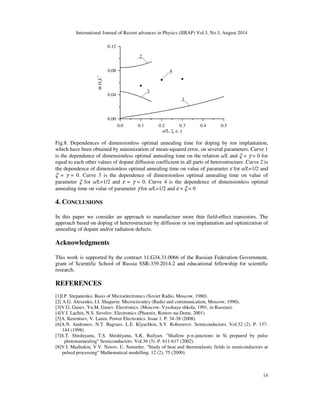Anzeige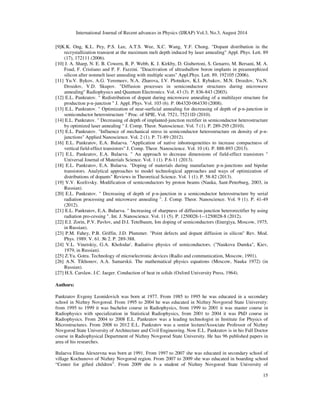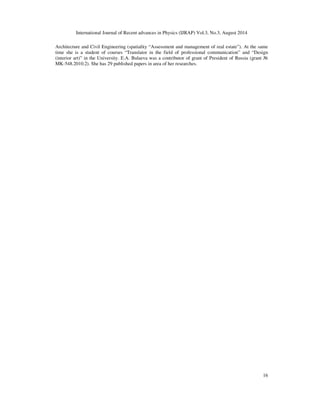Nächste SlideShare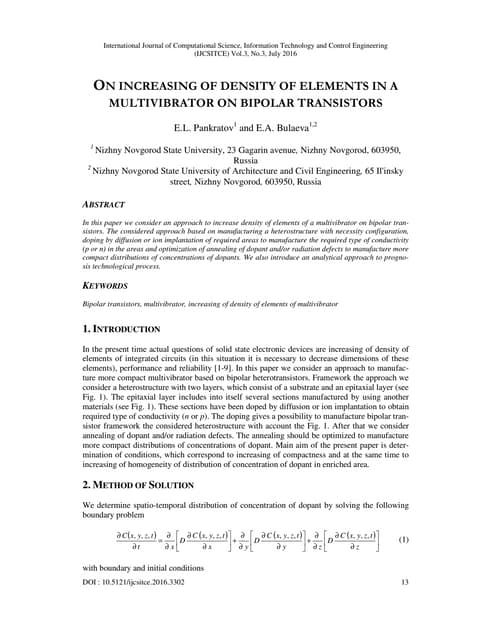ON INCREASING OF DENSITY OF ELEMENTS IN A MULTIVIBRATOR ON BIPOLAR TRANSISTORS
1 von 16
Anzeige

### OPTIMIZATION OF MANUFACTURE OF FIELDEFFECT HETEROTRANSISTORS WITHOUT P-NJUNCTIONS TO DECREASE THEIR DIMENSIONS

1. International Journal of Recent advances in Physics (IJRAP) Vol.3, No.3, August 2014 OPTIMIZATION OF MANUFACTURE OF FIELD-EFFECT HETEROTRANSISTORS WITHOUT P-N- JUNCTIONS TO DECREASE THEIR DIMENSIONS E.L. Pankratov1, E.A. Bulaeva2 1 Nizhny Novgorod State University, 23 Gagarin avenue, Nizhny Novgorod, 603950, Russia 2 Nizhny Novgorod State University of Architecture and Civil Engineering, 65 Il'insky street, Nizhny Novgorod, 603950, Russia ABSTRACT It has been recently shown, that manufacturing p-n-junctions, field-effect and bipolar transistors, thyristors in a multilayer structure by diffusion or ion implantation under condition of optimization of dopant and/or radiation defects leads to increasing of sharpness of p-n-junctions (both single p-n-junctions and p-n-junctions, which include into their system). In this situation one can also obtain increasing of homogeneity of dopant in doped area. In this paper we consider manufacturing a field-effect heterotransistor without p-n- junction. Optimization of technological process with using inhomogeneity of heterostructure give us possibility to manufacture the transistors as more compact. KEYWORDS Field-effect heterotransistors; Decreasing of Dimensions of Transistors 1.INTRODUCTION The development of electronics makes it necessary to reduce the size of integrated circuit elements and their discrete analogs [1-7]. To reduce the required sizes formed by diffusion and implantation of p-n-junctions and their systems (such transistors and thyristors) it could be used several approaches. First of all it should be considered laser and microwave types of annealing [8- 14]. Using this types of annealing leads to generation inhomogenous distribution of temperature gives us possibility to increase sharpness of p-n-junctions with simultaneous increasing of homogeneity of distribution of dopant concentration in doped area [8-14]. In this situation one can obtain more shallow p-n-junctions and at the same time to decrease dimensions of transistors, which include into itself the p-n-junctions. The second way to decrease dimensions of elements of integrated circuits is using of native inhomogeneity of heterostructure and optimization of annealing [12-18]. In this case one can obtain increasing of sharpness of p-n-junctions and at the same time increasing of homogeneity of distribution of dopant concentration in doped area [12- 18]. Distribution of concentration of dopant could be also changed under influence of radiation processing . In this situation radiation processing could be also used to increase sharpness of single p-n-junction and p-n-junctions, which include into their system [20,21]. In this paper we consider a heterostructure, which consist of a substrate and a multi-section epitaxial layer (see Figs. 1 and 2). Farther we consider manufacturing a field-effect transistor DOI : 10.14810/ijrap.2014.3301 1
2. International Journal of Recent advances in Physics (IJRAP) Vol.3, No.3, August 2014 without p-n-junction in this heterostructure. Appropriate contacts are presented in the Figs. 1 and 2. A dopant has been infused or implanted in areas of source and drain before manufacturing the contacts to produce required types of conductivity. Farther we consider annealing of dopant and/or radiation defects to infuse the dopant on required depth (in the first case) or to decrease quantity of radiation defects (in the second case). Annealing of radiation defects leads to spreading of distribution of concentration of dopant. To restrict the dopant diffusion into substrate and to manufacture more thin structure it is practicably to choose properties of heterostructure so, that dopant diffusion coefficient in the substrate should be as smaller as it is possible, than dopant diffusion coefficient in epitaxial layer [12-18]. In this case it is practicably to optimize annealing time [12-18]. If dopant did not achieve interface between layers of heterostructure during annealing of radiation defects it is practicably to choose addition annealing of dopant. Main aims of the present paper are (i) modeling of redistribution of dopant and radiation defects; (ii) optimization of annealing time. 2 Fig. 1. Heterostructure with a substrate and multi-section epitaxial layer. View from top Epitaxial layer Source Gate Drain Substrate Fig. 2. Heterostructure with a substrate and multi-section epitaxial layer. View from one side 2. Method of solution To solve our aims we determine spatio-temporal distribution of concentration of dopant. We determine the distribution by solving the second Fick’s law [1-4]
3. International Journal of Recent advances in Physics (IJRAP) Vol.3, No.3, August 2014 3 ( ) ( ) ¶ , , , , = C x t x D C x y z t ¶ t x ¶ C ¶ ¶ ¶ (1) with boundary and initial conditions ( ) 0 , 0 = C x t ¶ ¶ x= x , ( ) 0 , = C x t ¶ ¶ x=L x , C (x,0)=f (x). (2) Here C(x,t) is the spatio-temporal distribution of concentration of dopant; T is the temperature of annealing; D is the dopant diffusion coefficient. Value of dopant diffusion coefficient depends on properties of materials of heterostructure, speed of heating and cooling of heterostructure (with account Arrhenius law). Dependences of dopant diffusion on parameters could be approximated by the following function [22-25] ( ) ( ) , , , V x t V x t g C x t D D x T C L x V V g = + * 2 ( ) ( ) ( ) ( ) + + 2 1 * 2 1 , , 1 V V P x T , (3) where DL (x,T) is the spatial (due to native inhomogeneity of heterostructure) and temperature (due to Arrhenius law) dependences of dopant diffusion coefficient; P (x, T) is the limit of solubility of dopant; parameter g depends on properties of materials and could be integer in the following interval g Î[1,3] ; V (x,t) is the spatio-temporal distribution of concentration of radiation vacancies; V* is the equilibrium distribution of vacancies. Concentrational dependence of dopant diffusion coefficient has been described in details in . It should be noted, that using of doping of materials by diffusion leads to absents of radiation damage z1= z2= 0. We determine spatio-temporal distributions of concentrations of radiation defects by solving the following system of equations [23,24] ( ) ( ) ( ) ( ) ( ) ( ) ( ) ( ) I I V I I ( ) ( ) I x t ( ) I x t ¶ − ( ) ( ) ( ) − ( ) ( ) = − − = , , , , , k x T I x t V x t k x T V x t , V x t x , D x T V x t ¶ t x k x T I x t V x t k x T I x t x D x T t x V I V V V , , , , , , , , , 2 , , 2 , , ¶ ¶ ¶ ¶ ¶ ¶ ¶ ¶ ¶ ¶ (4) with initial r (x,0)=fr (x) (5a) and boundary conditions ( ) 0 , 0 = I x t ¶ ¶ x= x , ( ) 0 , = I x t ¶ ¶ x=L x , ( ) 0 , 0 = V x t ¶ ¶ x= x , ( ) 0 , = V x t ¶ ¶ x=L x , I(x,0)=fI (x), V(x,0)=fV (x). (5b)
4. International Journal of Recent advances in Physics (IJRAP) Vol.3, No.3, August 2014 Here r =I,V; I (x,t) is the spatio-temporal distribution of concentration of interstitials; Dr(x,T) is the diffusion coefficient of point radiation defects (vacancies and interstitials); terms V2(x,t) and I2(x,t) corresponds to generation of divacancies and diinterstitials; kI,V(x,T), kI,I(x,T) and kV,V(x,T) are parameters of recombination of point radiation defects and generation their simplest complexes (divacancies and diinterstitials). We determine spatio-temporal distributions of concentrations of divacancies FV (x,t) and diinterstitials FI 4 (x,t) by solving of the following system of equations [23,24] ( ) ( ) ( ) ( ) ( ) ( ) ( ) ( ) ( ) ( ) ( ) ( ) ( ) ( ) − + F F F = F − + = F F k x T V x t k x T V x t x t x t x D x T x t x t ¶ ¶ t x k x T I x t k x T I x t x D x T t x V V V V V V I I I I I I , , , , , , , , , , , , , , 2 , 2 , ¶ ¶ ¶ ¶ ¶ ¶ ¶ ¶ ¶ ¶ (6) with boundary and initial conditions ( ) 0 , 0 = I x t ¶ ¶ x= x , ( ) 0 , = I x t ¶ ¶ x=L x , ( ) 0 , 0 = V x t ¶ ¶ x= x , ( ) 0 , = V x t ¶ ¶ x=L x , I(x,0)=fI (x), V(x,0)=fV (x). (7) Here DFI(x,T) and DFV(x,T) are diffusion coefficients of divacancies and diinterstitials; kI(x,T) and kV (x,T) are parameters of decay of complexes of radiation defects. To determine spatio-temporal distributions of concentrations of point radiation defects we used recently introduced approach [16,18]. Framework the approach we transform approximations of diffusion coefficients of point radiation defects to the following form: Dr(x,T)=D0r[1+ergr(x,T)], where D0r is the average value of the diffusion coefficients, 0£er 1, |gr(x,T)|£1, r =I,V. We also transform parameters of recombination of point defects and generation their complexes in the same form: kI,V(x, T)=k0I,V[1+ eI,V gI,V(x,T)], kI,I(x,T)=k0I,I[1+ eI,I gI,I(x,T)] and kV,V(x,T) = k0V,V [1+eV,V gV,V(x,T)], where k0r1,r2 is the appropriate average values of the above parameters, 0£ eI,V1, 0£ eI,I 1, 0£eV,V 1, | gI,V (x,T)|£1, | gI,I(x,T)|£1, |gV,V(x,T)|£1. We introduce the following dimensionless variables: 2 2 w = , ( ) ( ) * , , 0 0 D D t L I V J = , I V I V L k D D0 , 0 0 ~ I x t = I x t I , c = x/Lx, h = y/Ly, f = ~ V x t =V x t V , , I V L k D D0 0 0 z/Lz, ( ) ( ) * , , 2 r r r W = . The introduction transforms Eq.(4) and conditions (5) in the following form ( ) [ ( )] ( ) [ ( )] ( ) [ ( )] ( ) ( ) ( ) [ ( )] ( ) [ ( )] ( ) c J I D 0 I w e c c J c J − + ¶ g T I V V c J D − [ + ( ) ] ( ) ( ) −W + −
5. c J ¶ ¶ + ¶ ¶ = ¶ ¶ −W + −
6. ¶ ¶ + ¶ = ¶ ¶ 0 V w e c c J c J e c c J c J c e c J c e c c J c e c J c , ~ , ~ 1 , , ~ 1 , , ~ 1 , , ~ , ~ , ~ 1 , , ~ 1 , , ~ 1 , , ~ , , 2 , , 0 0 , , 2 , , 0 0 g T I V g T V V g T D D g T I I g T D D I V I V V V V V V V V I V I V I V I I I I I I I I V (8)
7. International Journal of Recent advances in Physics (IJRAP) Vol.3, No.3, August 2014 5 ( ) 0 ~ , 0 = r c J ¶ ¶ c = c , ( ) 0 ~ , 1 = r c J ¶ ¶ c = c r c J r f = (9) , ( ) ( c , J ) * ~ , r We determine solutions of Eqs.(8) and conditions (9) framework recently introduce [16,18] approach as the power series ¥ ~ , ~ , ( ) = W ( ) i j k r c J e w r c J r r . (10) = ¥ = ¥ 0 0 =0 i j k ijk Substitution of the series (10) into Eq.(8) and conditions (9) gives us possibility to obtain equations for the zero-order approximations of concentrations of point defects (c ,J ) ~ 000 I and ~ V (c ,J ) , corrections for them (c ,J ) 000 ~ ijk I and (c ,J ) ~ ijk V (i ³1, j ³1, k ³1) and conditions for all ~ ijk I and (c ,J ) functions (c ,J ) ~ ijk V (i ³0, j ³0, k ³0) ( c J ) ( ) I D 000 0 c J I V ( ) ( ) ¶ ¶ c J V D 000 0 = ¶ ¶ ¶ ¶ = ¶ ¶ c J 2 000 2 V 0 2 000 2 0 , ~ , ~ , ~ , ~ c J c J V D I D I ; ( ) ( ) ( ) ( ) ¶ − c J c J c J I D 0 I D 00 0 i I i I ( ) ( ) ( ) ( ) ¶ ¶ ¶ ¶ V D i V + ¶ ¶ V D 00 0 i V = ¶ ¶ ¶ ¶ ¶ + ¶ ¶ = ¶ ¶ − c J c c c J 0 c c c J J c c c c J , ~ , , ~ , ~ , ~ , , ~ , ~ 100 0 2 00 2 0 100 0 2 00 2 0 i V I I i I V V V g T D D I g T D D , i ³1; ( ) ( ) [ ( )] ( ) ( ) ( ) ( ) [ ( )] ( ) ( ) − + ¶ ¶ I D 010 0 V D 010 0 = ¶ ¶ − + ¶ ¶ = ¶ ¶ e c c J c J c J c J c c J c J J e c c J c J c J , ~ , ~ 1 , , ~ , ~ , ~ , ~ 1 , , ~ , ~ 2 , , 000 000 010 2 I V 0 2 , , 000 000 010 2 0 g T I V V D g T I V I D I V I V I I V I V V ; ( ) ( ) − c , J [ ( )] [ ( ) ( ) ( ) ( )] ¶ c J I D 020 0 I ( ) ( ) ¶ V D ¶ c , J 020 = 0 − ¶ ¶ − [ + ( ) ] [ ( ) ( ) + ( ) ( ) ] − + + ¶ = ¶ ¶ e c c J c J c J c J c c J J e c c J c J c J c J c J , ~ , ~ , ~ , ~ 1 , ~ , ~ , ~ , ~ , ~ , ~ 1 , ~ , ~ , , 010 000 000 010 2 020 2 0 , , 010 000 000 010 2 020 2 0 g T I V I V V D g T I V I V I D I V I V I V I V I V V ;
8. International Journal of Recent advances in Physics (IJRAP) Vol.3, No.3, August 2014 6 ( ) ( ) [ ( )] ( ) ( ) ( ) [ ( )] ( ) − + ¶ ¶ I D 001 0 V D 001 0 = ¶ ¶ − + ¶ ¶ = ¶ ¶ e c c J c J c J c c J c J J e c c J c J , ~ 1 , , ~ , ~ , ~ 1 , , ~ , ~ 2 2 , , 000 001 2 I V 0 2 2 , , 000 001 2 0 g T V V D g T I I D I I I I I I I I I V ; ( ) ( ) ( ) ( ) ¶ c J c c c J 0 I D I c c 010 0 I g T D [ ( )][ ( ) ( ) ( ) ( )] c J I D 110 0 I ( ) ( ) ( ) ( ) V c J D ¶ V c J 0 V g c T 010 c D c c − 0 − [ + ( ) ] [ ( ) ( ) + ( ) ( ) ] ¶ ¶ ¶ + ¶ ¶ V D 110 0 = ¶ ¶ − + + − ¶ ¶ ¶ + ¶ ¶ = ¶ ¶ e c c J c J c J c J c J J e c c J c J c J c J J , ~ , ~ , ~ , ~ 1 , , ~ , , ~ , ~ , ~ , ~ , ~ , ~ 1 , , ~ , , ~ , ~ , , 100 000 000 100 2 110 2 V 0 , , 100 000 000 100 2 110 2 0 g T V I V I D g T I V I V D V V V V V I I I I I I I V V ; ( ) ( ) [ ( )] ( ) ( ) ( ) ( ) [ ( )] ( ) ( ) − + ¶ ¶ I D 002 0 V D 002 0 = ¶ ¶ − + ¶ ¶ = ¶ ¶ e c c J c J c J c J c c J c J J e c c J c J c J , ~ , ~ 1 , , ~ , ~ , ~ , ~ 1 , , ~ , ~ 2 , , 001 000 002 2 I V 0 2 , , 001 000 002 2 0 g T V V V D g T I I I D I I I I I I I I I V ; ( ) ( ) ( ) ( ) c J c I [ ( )] ( ) ( ) ¶ c J c J I D 0 I D 101 0 I I ( ) ( ) ( ) ( ) − − [ + ( ) ] ( ) ( ) ¶ ¶ ¶ ¶ V D + ¶ ¶ V D 101 0 = ¶ ¶ − + − ¶ ¶ ¶ + ¶ ¶ = ¶ ¶ c J c V e c c J c J c c J 0 V c c c J J e c c J c J c c c J , ~ , ~ 1 , , ~ , , ~ , ~ , ~ , ~ 1 , , ~ , , ~ , ~ 000 100 001 0 2 101 2 V 0 100 000 001 0 2 101 2 0 g T I V g T D D g T I V g T D D V V V I I I I I V V ; ( ) ( ) [ ( )] ( ) ( ) − + − e g c T I c J I c J 2 , , 000 010 [ ( )] ( ) ( ) c J c J I D 011 0 I ( ) ( ) [ ( )] ( ) ( ) [ ( ) ] ( ) ( ) − + − e g c T V c J V c J 2 , , 000 010 − + ¶ ¶ V D 011 0 = ¶ ¶ − + ¶ ¶ = ¶ ¶ e c c J c J c J c c J J e c c J c J c J , ~ , ~ 1 , , ~ , ~ 1 , , ~ , ~ , ~ , ~ 1 , , ~ , ~ 1 , , ~ , ~ , , 000 001 011 2 V 0 , , 001 000 011 2 0 g T I V V D g T I V I D I V I V V V V V I I V I V I I I I V ; ( ) 0 ~ , 0 = r c J ¶ ¶ x= ijk c , ( ) 0 ~ , 1 = r c J ¶ ¶ x= ijk c , (i ³0, j ³0, k ³0); r~ ( c ,0 ) = f ( c ) r * , ~ ( c , 0 ) = 0 (i ³1, j ³1, k ³1). 000 r ijk r
9. International Journal of Recent advances in Physics (IJRAP) Vol.3, No.3, August 2014 Solutions of the obtained equations could be obtained by standard approaches (see, for example, [26,27]). The solutions with account appropriate boundary and initial conditions could be written as ~ 010 000 000 010 × + ; 7 ¥ ( ) = + ( ) ( ) r c J c J r r , =1 000 1 2 ~ , n n n F c e L L where ( ) ( ) nI V I e n D D0 0 2 2 J = exp −p J , ( ) ( ) nV I V e n D D0 0 2 2 J = exp −p J , cn(c) = cos (p n c), 1 = ( ) ( ) * cos 0 1 F nu f u d u nr nr p r ; ( ) D 0 I ( ) ( ) J ( ) ( ) ( ) I ( u ) ( ) ( ) ( ) ( ) ( ) ( ) ( ) V u ¶ ¶ 0 V D = − − ¶ ¶ = − − ¥ = − ¥ = − 1 0 1 0 100 0 00 1 0 1 0 100 0 00 , ~ , 2 , ~ , ~ , 2 , ~ n i n nV nV n V I i n i n nI nI n I V i d u d u nc e e s u g u T D V d u d u nc e e s u g u T D I J t t c J p c J t t t c J p c J t , i ³1, where sn(c) = sin (p n c); ¥ J ( ) = − ( ) ( ) (− ) ( )[ + ( )] ( ) ( ) r c J c f J t e t , t t ; 010 r r , , 000 000 =1 0 1 0 ~ , ~ ~ , 2 , 1 , n n n n I V I V c e e c u g u T I u V u d u d D ¥ J ( )= − ( ) ( ) (− ) ( )[ + ( )] × 020 ~ , 2 1 , I c e e c u g u T r r r c J c J t e =1 0 1 0 , , 0 0 n n n n I V I V V D [(~ ~ ~ I c , J )V (c , J ) I (c , J )V (c ,J )]d u dt J ( ) ¥ = − ( ) ( ) (− ) ( ) [ + ( )] ( ) 001 , , 000 ~ , 2 1 , ~ , r r r r r r r c J c J t e r t t ; =1 0 1 0 2 n n n n n c e e c u g u T u d u d J ( ) ¥ = − ( ) ( ) (− ) ( )[ + ( )] ( ) ( ) 002 , , 001 000 ~ , 2 1 , ~ , ~ , r r r r r r r c J c J t e r t r t t ; =1 0 1 0 n n n n n c e e c u g u T u u d u d ( ) ( ) ( ) ( ) 1 ( ) ( ) I ( u t ) ( ) t − c × D 0 I c J p c J t 010 d u d c u nc e e s u g u T 1 0 0 1 D ( ) ( ) ( )[ ( ) ( ) ( ) ( )][ ( )] × − + + J t t t t t e t nI nI n I V I V ( ) ( ) ( ) ( ) ( ) ( ) ( ) ( ) 1 V u t ( ) ( ) ( ) [ ( ) ( ) ( ) ( ) ] [ ( ) ] − × D 0 V c J p c J t 010 d u d c u nc e e s u g u T 1 0 0 1 D × − + + ¶ ¶ = − − ¶ ¶ = − − ¥ t c = ¥ = ¥ = ¥ = J J J J J t t t t t e t 0 1 0 000 100 100 000 , , 0 110 0 1 0 100 000 000 100 , , 0 110 , 1 , ~ , ~ , ~ , ~ 2 , ~ , 2 , ~ , 1 , ~ , ~ , ~ , ~ 2 , ~ , 2 , ~ e e c u I u V u I u V u g u T d u d V e e c u I u V u I u V u g u T d u d I nV nV n I V I V n n n n nV nV n V I n n n n nI nI n I V ;
10. International Journal of Recent advances in Physics (IJRAP) Vol.3, No.3, August 2014 8 ( ) ( ) ( ) ( ) ( ) ( ) I ( u t ) − D 0 c J p c J t n nc e e s u g u T n nI nI n I I V d u d u D ( ) ( ) ( ) ( )[ ( )] ( ) ( ) − − + t c J t e t t t ( ) ( ) ( ) ( ) ( ) ( ) ( ) V u t ( ) ( ) ( ) ( ) [ ( ) ] ( ) ( ) − D 0 c J p c J t n nc e e s u g u T n nV nV n V V I D − − + ¶ ¶ = − − ¶ ¶ = − − ¥ = ¥ = ¥ = ¥ = 1 0 1 0 u , , 000 100 1 0 1 0 001 0 101 1 0 1 0 , , 100 000 1 0 1 0 001 0 101 d u d , ~ , ~ 2 1 , , ~ , 2 , ~ , ~ , ~ 2 1 , , ~ , 2 , ~ n n nV nV n I V I V n n nI nI n I V I V t c e e c u g u T I u V u d u d V c e e c u g u T I u V u d u d I J J J J c J t e t t t ; ( ) ¥ ( ) ( ) J ( ) ( ){[ ( )] ( ) ( ) = − − + + c J c J t e t t I c e e c u g u T I u I u 011 , , 000 010 [ ( )] ( ) ( )} + + = e g u T I u t V u t d u d t I V I V ( ) ( ) ( ) ( ) ( ){[ ( )] ( ) ( ) V c J = − c c e J e − t c u + e g u T V u t V u t + 011 , , 000 010 + [ + ( ) ] ( ) ( )} ¥ = , . ~ , ~ 1 , , ~ , ~ , 2 1 , ~ , ~ , ~ 1 , , ~ , ~ , 2 1 , ~ , , 001 000 1 0 1 0 , , 001 000 1 0 1 0 e t t t J g u T I u V u d u d I V I V n n nV nV n V V V V n n nI nI n I I I I Farther we determine spatio-temporal distribution of concentration of complexes of point radiation defects. To calculate the distribution we transform approximation of diffusion coefficient to the following form: DFr(x,T)=D0Fr[1+eFrgFr(x,T)], where D0Fr are the average values of diffusion coefficient. After this transformation Eqs.(6) transforms to the following form ( ) [ ( )] ( ) ( ) ( ) ( ) ( ) ( ) [ ( )] ( ) ( ) ( ) ( ) ( ) + −
11. ¶ F F F F ¶ = + F + −
12. F = + F F F F k x T V x t k x T V x t x t x t x g x T x D x t t x t k x T I x t k x T I x t x g x T x D t V V V V V V V V I I I I I I I I , , , , , 1 , , , , , , , 1 , , 2 0 , 2 0 , ¶ ¶ e ¶ ¶ ¶ ¶ ¶ e ¶ ¶ ¶ Let us determine solutions of the above equations as the following power series ¥ F ( )= F ( ) x , t e i x , t . (11) r r r = F 0 i i Substitution of the series (11) into Eqs.(6) and conditions for them gives us possibility to obtain equations for initial-order approximations of concentrations of complexes, corrections for them and conditions for all equations in the following form ( ) ( ) ( ) ( ) ( ) ( ) ( ) ( ) ( ) ( ) ( ) ( ) + − F = F + − F = F F F k x T V x t k x T V x t 0 x x t D t x t k x T I x t k x T I x t x x t D t x t V V V V V V I I I I I I , , , , , , , , , , , , 2 2 , 2 0 0 2 2 , 0 2 0 0 ¶ ¶ ¶ ¶ ¶ ¶ ¶ ¶ ;
13. International Journal of Recent advances in Physics (IJRAP) Vol.3, No.3, August 2014 9 ( ) ( ) F ( ) ( ) ( ) ( ) F ( ) ( ) + F = F + F = F − ¶ ¶ F F F − F F F x x t g x T x D x x t D t x t x x t g x T x D x x t D t x t V i V V V i V V i I i I I I i I I i ¶ ¶ ¶ ¶ ¶ ¶ ¶ ¶ ¶ ¶ ¶ ¶ ¶ ¶ , , , , , , , , 1 2 0 2 0 1 2 0 2 0 , i³1; ( ) x t r 0 , , 0 = ¶ ¶F x= i x ( ) x t r 0 , i³0; Fr0(x,0)=fFr (x), Fri(x,0)=0, i³1. , = ¶ ¶F x=L i x Solutions of the above equations could be written as ¥ F ( ) = + ( ) ( )+ = F F 1 0 1 2 , n n n F c x e t L L x t r r r ¥ + ( ) ( ) (− ) ( )[ ( ) ( )− ( ) ( )] = F F 1 0 0 2 , , , , , 2 n t L n n n n I I I nc x e t e c u k u T I u k u T I u d u d L t t t t r r , where ( ) ( 2 ) p F F = − , = ( ) ( ) F F e t exp 2 n 2 D t L n r 0 r L n n F c u f u d u r r 0 , cn(x) = cos (p n x/L); p ¥ r ( ) ( ) ( ) ( ) ( ) ( ) ( ) F r r r r , i³1, F = − − ¶ t x t t = − F F F 1 0 0 1 2 , , 2 , n t L I i i n n n n d u d u u nc x e t e s u g u T L ¶ t where sn(x) = sin (p n x/L) Spatio-temporal distribution of concentration of dopant we determine framework the recently introduced approach. Framework the approach we transform approximation of dopant diffusion coefficient to the following form: DL(x,T)=D0L[1+eLgL(x,T)], where D0L is the average value of dopant diffusion coefficient, 0£eL 1, |gL(x,T)|£1. Farther we determine solution of the Eq.(1) as the following power series ¥ ( ) = ( ) C x , t e i x j C x , t . L = ¥ 0 =1 i j ij Substitution of the series into Eq.(1) and conditions (2) gives us possibility to obtain zero-order approximation of dopant concentration C00(x,t), corrections for them Cij(x,t) (i ³1, j ³1) and conditions for all functions. The equations and conditions could be written as ( ) ¶ ( ) 00 , , C x t 2 00 2 0 x D C x t t L ¶ = ¶ ¶ ; ( ) ( ) ( ) ( ) 0 , i ³1; ¶ − i , , , 10 C x t i C x t ¶ ¶ ¶ ¶ + C x t ¶ ¶ = ¶ x g x T x D x D t L L i L , 2 0 0 2 0 ( ) ( ) g ( ) , , C x , t 00 00 ( ) ( ) C x t ¶ ¶ ¶ ¶ + C x t ¶ ¶ = C x t ¶ ¶ x P x T x D x D t L L , , 2 0 01 2 0 01 g ;
14. International Journal of Recent advances in Physics (IJRAP) Vol.3, No.3, August 2014 10 ( ) ( ) ¶ g − , , 00 ( ) ( ) ( ) ( ) + C x t ¶ ¶ ¶ ¶ + C x t ¶ ¶ = C x t ¶ x C x t P x T C x t x D x D t L L , , , , 1 00 2 0 01 02 2 0 02 g ( ) ( ) ( ) g , 00 01 C x t ¶ ¶ ¶ ¶ + x C x t P x T x D L , , 0 g ; ( ) ( ) ( ) ( ) ¶ g − C x t , , 00 ( ) ( ) + C x t ¶ ¶ ¶ ¶ + C x t ¶ ¶ = C x t ¶ x P x T C x t x D x D t L L , , , , 1 00 2 0 10 11 2 0 11 g ( ) ( ) ( ) ( ) ( ) g D L L L C x t ¶ ¶ ¶ ¶ + C x t ¶ ¶ ¶ ¶ + x g x T x D x C x t P x T x , , , , , 01 0 00 10 0 g ; ( ) 0 , 0 = x= C x t ij ¶ x ¶ , ( ) 0 , = x=L C x t ij ¶ x ¶ , i ³0, j ³0; C00(x,0)=fC (x), Cij(x,0)=0, i ³1, j ³1. Solutions of the equations with account appropriate boundary and initial conditions could be obtained by standard approaches (see, for example, [26,27]). The solutions are ¥ ( ) = + ( ) ( ) C x t , =1 00 1 2 , n nC nC F c x e t L L where ( ) ( 2 ) 2 2 e t exp n D t L nC C = −p , = ( ) ( ) 0 L nC n C F c u f u d u 0 ; ( ) ¥ ( ) ( ) ( ) ( ) ( ) C ( u ) C x t t ¶ ¶ = − − = − 1 0 0 10 0 2 , , 2 , n t L i i nC n nC nC n L d u d u nF c x e t e s u g u T L t t p , i ³1; 2 g ( ) ( ) ( ) ( ) ( ) C ( u , ) C x t t ( ) ( ) ¥ C u ¶ ¶ = − − =1 0 0 00 00 01 2 , , , n t L nC n nC nC n d u d u P u T nF c x e t e s u L t t t p g ; g ( ) ( ) ( ) ( ) ( ) ( ) C ( u ) C x t t ( ) ( ) ¥ C u − ¶ ¶ = − − = − 1 0 0 00 1 00 02 2 01 , , , , 2 , n t L nC n nC nC n d u d u P u T nF c x e t e s u C u L t t t t p g g ( ) ( ) ( ) ( ) ( ) 2 C u , ( ) ( ) ¥ C u ¶ ¶ − − =1 0 0 00 01 2 , , n t L nC n nC nC n d u d u P u T n F c x e t e s u L t t t t p g ; ( ) ( ) ( ) ( ) ( ) ( ) C ( u ) − × ¶ ¶ = − − ¥ = ¥ 2 = 1 1 0 0 01 11 2 , 2 , 2 , n nC n t L nC n nC nC n L nF L d u d u nF c x e t e s u g u T L C x t p t t t p ( ) ( ) ( ) ( ) ( ) C g u , ( ) − ( ) ( ) ( − ) × c x e t e s u t ( ) C u ¶ ¶ × − ¥ =1 0 2 0 0 00 10 , 2 , n t nC n nC nC t L n nC nC n nF c x e t e L d u d u P u T p t t t t g ( ) ( ) ( ) ( ) ( ) t C u t ¶ ¶ × g − L C u n d u d u P u T s u C u 0 00 1 00 10 , , , , t t g . Analysis of spatio-temporal distributions of concentrations of dopant and radiation defects has been done analytically by using the second-order approximations framework recently introduced power series. The approximation is enough good approximation to make qualitative analysis and to obtain some quantitative results. All obtained analytical results have been checked by comparison with results, calculated by numerical simulation.
15. International Journal of Recent advances in Physics (IJRAP) Vol.3, No.3, August 2014 11 3. Discussion In this section based on obtained in the previous section relations we analysed dynamics of redistribution of dopant, which was infused in heterostructure from Figs. 1 and 2 by diffusion or ion implantation, during annealing of the dopant and/or radiation defects. We take into account radiation damage during consideration dopant redistribution after ion doping of heterostructure. The Fig. 3 shows typical distributions of concentrations of infused dopant in heterostructure in direction, which is perpendicular to interface between epitaxial layer and substrate. The distributions have been calculated under condition, when value of dopant diffusion coefficient in epitaxial layer is larger, than value of dopant diffusion coefficient in substrate. Analogous distributions of dopant concentrations for ion doping are presented in Fig. 4. The Figs. 3 and 4 shows, that presents of interface between layers of heterostructure under above condition gives us possibility to obtain more thin field-effect transistor. At the same time one can find increasing of homogeneity of dopant distribution in doped area. Fig.3. Distributions of concentration of infused dopant in heterostructure from Figs. 1 and 2 in direction, which is perpendicular to interface between epitaxial layer substrate. Increasing of number of curve corresponds to increasing of difference between values of dopant diffusion coefficient in layers of heterostructure under condition, when value of dopant diffusion coefficient in epitaxial layer is larger, than value of dopant diffusion coefficient in substrate Epitaxial layer Substrate 0 L/4 L/2 3L/4 L x 2.0 1.5 1.0 0.5 0.0 C(x,Q) 2 3 4 1 Fig.4. Distributions of concentration of implanted dopant in heterostructure from Figs. 1 and 2 in direction, which is perpendicular to interface between epitaxial layer substrate. Curves 1 and 3
16. International Journal of Recent advances in Physics (IJRAP) Vol.3, No.3, August 2014 where y (x) is the approximation function, which presented in Figs. 5 and 6 as curve 1. We determine optimal annealing time by minimization mean-squared error (12). Dependences of optimal annealing time on parameters for diffusion a ion types of doping are presented on Figs. 7 and 8. Optimal annealing time, which corresponds to ion doping, is smaller, than the same time for doping by diffusion. Reason of this difference is necessity to anneal radiation defects. Optimization of annealing time for ion doping of materials should be done only in this case, when dopant did not achieves interface between layers of heterostructure during annealing of radiation defects. C(x,Q) 12 2+Ly corresponds to annealing time Q = 0.0048(Lx 2+Lz 2)/D0. Curves 2 and 4 corresponds to 2+Ly annealing time Q = 0.0057(Lx 2+Lz 2)/D0. Curves 1 and 2 corresponds to homogenous sample. Curves 3 and 4 corresponds to heterostructure under condition, when value of dopant diffusion coefficient in epitaxial layer is larger, than value of dopant diffusion coefficient in substrate 0 Lx Fig.5. Spatial distributions of concentration of infused dopant in heterostructure from Figs. 1 and 2. Curve 1 is the idealized distribution of dopant. Curves 2-4 are the real distributions of dopant for different values of annealing time. Increasing of number of curve correspondsincreasing of annealing time It should be noted, that in this situation optimization of annealing time required. Reason of this optimization is following. If annealing time is small, dopant did not achieve interface between layers of heterostructure. In this situation inhomogeneity of dopant concentration increases. If annealing time is large, dopant will infused too deep. Optimal annealing time we determine framework recently introduce approach [12-18,19,20]. Framework the criterion we approximate real distribution of dopant concentration by stepwise function (see Figs. 5 and 6). Farther we determine the following mean-squared error between real distribution of dopant concentration and the stepwise approximation L C x x d x L = [ ( Q)− ( )] U 0 , 1 y , (12) 2 1 3 4
17. International Journal of Recent advances in Physics (IJRAP) Vol.3, No.3, August 2014 0 L x C(x,Q) 1 2 3 4 Fig.6. Spatial distributions of concentration of implanted dopant in heterostructure from Figs. 1 and 2. Curve 1 is the idealized distribution of dopant. Curves 2-4 are the real distributions of dopant for different values of annealing time. Increasing of number of curve corresponds to 13 increasing of annealing time 3 2 4 1 0.0 0.1 0.2 0.3 0.4 0.5 a/L, x, e, g 0.5 0.4 0.3 0.2 0.1 0.0 Q D0 L-2 Fig. 7. Dependences of dimensionless optimal annealing time for doping by diffusion, which have been obtained by minimization of mean-squared error, on several parameters. Curve 1 is the dependence of dimensionless optimal annealing time on the relation a/L and x = g = 0 for equal to each other values of dopant diffusion coefficient in all parts of heterostructure. Curve 2 is the dependence of dimensionless optimal annealing time on value of parameter e for a/L=1/2 and x = g = 0. Curve 3 is the dependence of dimensionless optimal annealing time on value of parameter x for a/L=1/2 and e = g = 0. Curve 4 is the dependence of dimensionless optimal annealing time on value of parameter g for a/L=1/2 and e = x = 0
18. International Journal of Recent advances in Physics (IJRAP) Vol.3, No.3, August 2014 3 2 4 1 0.0 0.1 0.2 0.3 0.4 0.5 a/L, x, e, g 0.12 0.08 0.04 0.00 Q D0 L-2 Fig.8. Dependences of dimensionless optimal annealing time for doping by ion implantation, which have been obtained by minimization of mean-squared error, on several parameters. Curve 1 is the dependence of dimensionless optimal annealing time on the relation a/L and x = g = 0 for equal to each other values of dopant diffusion coefficient in all parts of heterostructure. Curve 2 is the dependence of dimensionless optimal annealing time on value of parameter e for a/L=1/2 and x = g = 0. Curve 3 is the dependence of dimensionless optimal annealing time on value of parameter x for a/L=1/2 and e = g = 0. Curve 4 is the dependence of dimensionless optimal annealing time on value of parameter g for a/L=1/2 and e = x = 0 14 4. CONCLUSIONS In this paper we consider an approach to manufacture more thin field-effect transistors. The approach based on doping of heterostructure by diffusion or ion implantation and optimization of annealing of dopant and/or radiation defects. Acknowledgments This work is supported by the contract 11.G34.31.0066 of the Russian Federation Government, grant of Scientific School of Russia SSR-339.2014.2 and educational fellowship for scientific research. REFERENCES I.P. Stepanenko. Basis of Microelectronics (Soviet Radio, Moscow, 1980).  A.G. Alexenko, I.I. Shagurin. Microcircuitry (Radio and communication, Moscow, 1990). V.G. Gusev, Yu.M. Gusev. Electronics. (Moscow: Vysshaya shkola, 1991, in Russian). V.I. Lachin, N.S. Savelov. Electronics (Phoenix, Rostov-na-Donu, 2001). A. Kerentsev, V. Lanin. Power Electronics. Issue 1. P. 34-38 (2008). A.N. Andronov, N.T. Bagraev, L.E. Klyachkin, S.V. Robozerov. Semiconductors. Vol.32 (2). P. 137- 144 (1998). S.T. Shishiyanu, T.S. Shishiyanu, S.K. Railyan. Shallow p-n-junctions in Si prepared by pulse photonannealing Semiconductors. Vol.36 (5). P. 611-617 (2002). V.I. Mazhukin, V.V. Nosov, U. Semmler. Study of heat and thermoelastic fields in semiconductors at pulsed processing Mathematical modelling. 12 (2), 75 (2000).